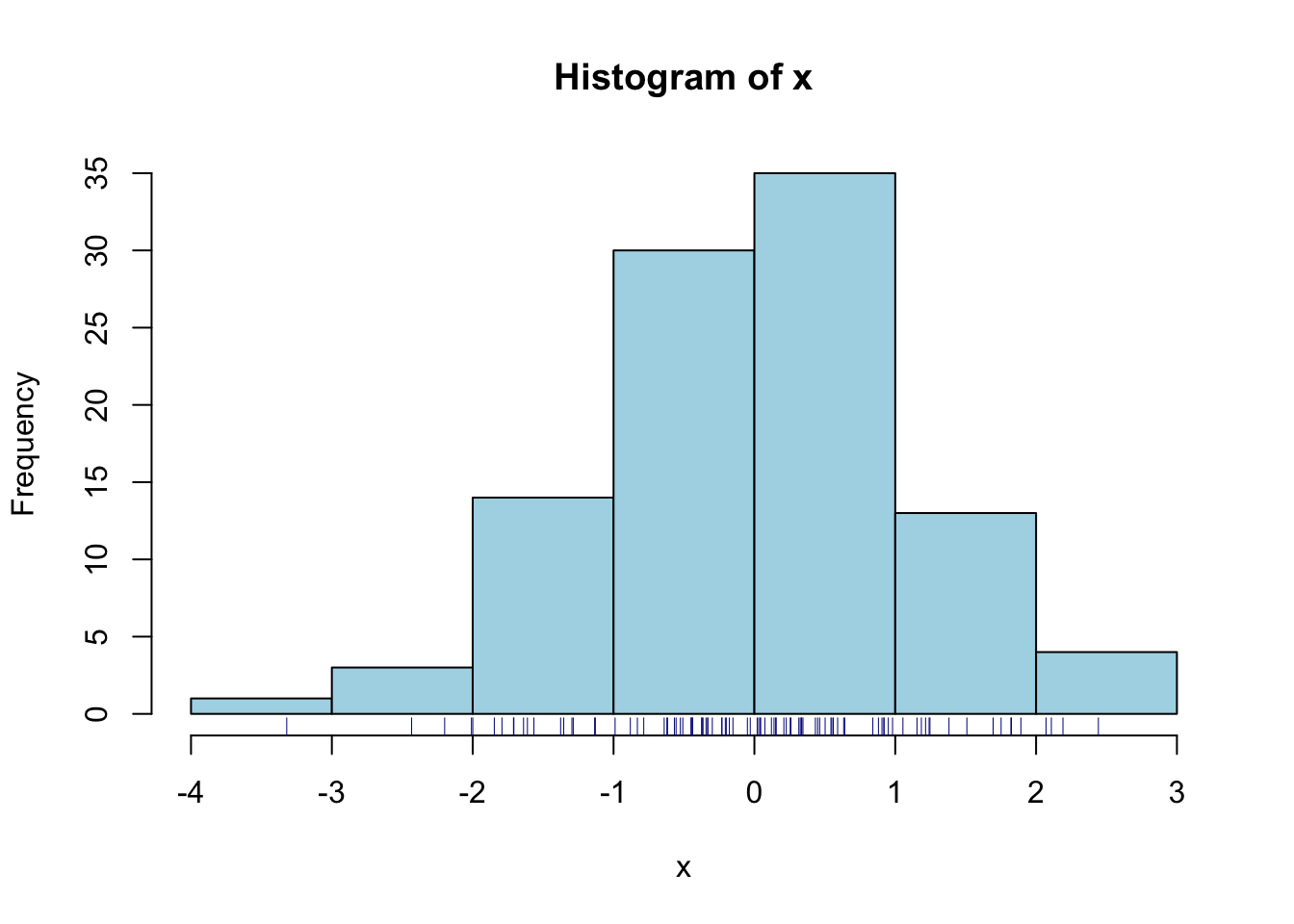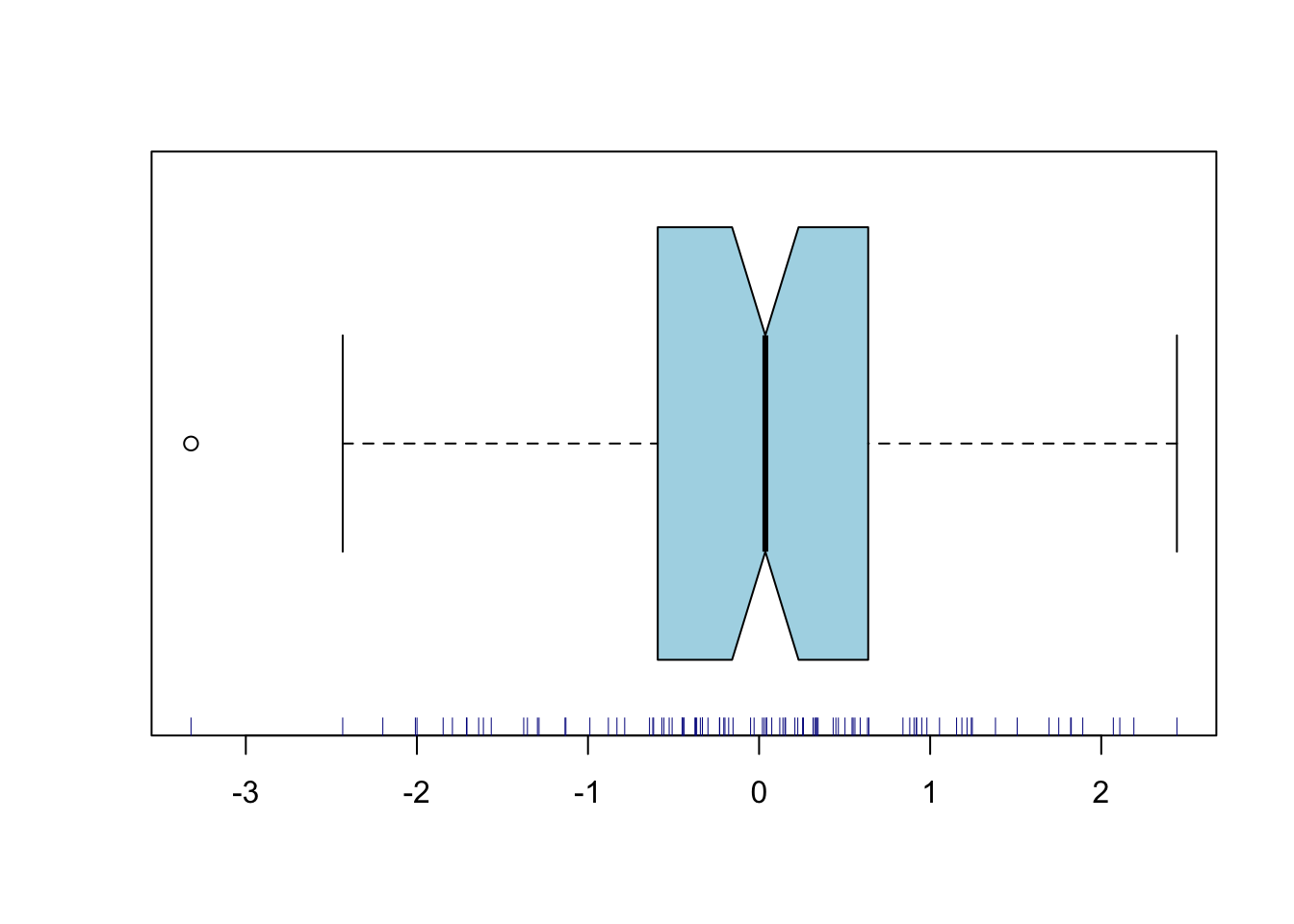# Chapter 4 Exploratory data analyses

R is more graphically oriented than most other statistical packages; it relies more on plots and figures for initial exploratory data analysis. Numerical summaries are of course also available.

## 4.1hist

This command produces a histogram. There is a useful optional argument breaks to specify the number of bins (bars), or a vector of breaks between bins.

## 4.2plot

The default version of this command produces a scattergram of two variables. If you enter just one variable, then the index numbers of the observations are used on the horizontal axis, and the values on the vertical axis. Useful arguments are title, and xlab and ylab for axis labels. In addition, you can use a third variable to vary the plot symbols.

## 4.3rug

This command produces tick marks at the actual data values, yielding the visual effect of a rug. This is useful in combination with a scattergram or histogram. Try it out, with the following commands:7

x<-rnorm(100)
hist(x,col="lightblue"); rug(x,col="darkblue")## 4.4boxplot

This yields a boxplot summary (Tukey 1977) of one variable. You can also specify the dependent and independent variable, with argument dv~iv1; this will produce multiple boxplots for the dependent variable, broken down by the independent variable(s). Two useful arguments for this command are:
notch=T to give additional information about the distribution, and
varwidth=T to scale the width of the boxes to the numbers of observations.

boxplot( x, col="lightblue", horizontal=TRUE, notch=TRUE, varwidth=TRUE )
rug(x, col="darkblue" )## 4.5qqnorm

This produces a quantile–quantile (QQ) plot. This plots the observed quantiles against the expected quantiles if the argument variable would be distributed normally. If the variable is indeed distributed normally, then the data should fall on an approximately straight line. Deviations of this line indicate deviations from normality. You can also add the expected line with qqline.

## 4.6summary

This command produces a numerical summary of the argument variable. However it does not supply standard measures of variability. We often need

## 4.7var

to compute the variance of the argument variable. Related functions are sd to compute standard deviation, cov to compute the covariance between two variables, and cor to compute their correlation.

## 4.8length

returns the length of the argument variable, i.e. the number of observations in that vector. This is useful for checking the number of data, as a preliminary for further analyses.

valid.n <- function(x) {
length(x)-length(which(is.na(x)))
}

In the last command above, we have programmed a new function valid.n, using standard functions provided with R.

## 4.9unique

returns a vector containing the (unsorted) unique values of the argument variable, without duplicates. This is also useful for checking your data.

x <- c(3,3,3,1,1,2); unique(x); sort(unique(x))
##  3 1 2
##  1 2 3

## 4.10table

returns a frequency table, i.e. reports the number of observations for each (combination of) value(s) of one or more variables. This again is helpful for checking your data, to inspect the numbers of observations per cell (see also 3.2). Tables can be one-dimensional (see example below) or multidimensional. It is also possible to make a frequency table of a frequency table!

x <- rep(1:3,each=7); table(x) # three cells, each 7 observations, N=21
## x
## 1 2 3
## 7 7 7
table( table(x) ) # ok: all three cells have 7 observations, N=21
##
## 7
## 3

A useful trick: if all cells should have the same number of observations, then the table-of-table should contain a single number representing the number of cells.

x[ sample(1:3*7, size=2) ] <- NA # replace 2 out of 21 obs by NA
table( table(x) ) # not ok: not all three cells have 7 observations, N<21
##
## 6 7
## 2 1

The lattice package (see Chapter 8) contains additional functions for higher-order data visualization: xyplot, histogram, densityplot, cloud, and more. For more information, enter the command help(lattice).

Now that we have obtained some insightful figures, we might want to include these in our documents. The best procedure in Rstudio is to go to the Plots tab in the lower right pane, and select Export > Save as image... or Export > Save as PDF.... Figures in PDF format (extension pdf) are easy to include in LaTeX documents, and are best for publication. Other formats (e.g. png) are easy to include in LaTeX, MS Word and web documents.

### References

Tukey, John W. 1977. Exploratory Data Analysis. Addison-Wesley.

1. The semicolon separates multiple R commands on a single line of input.